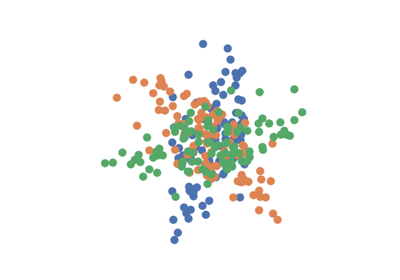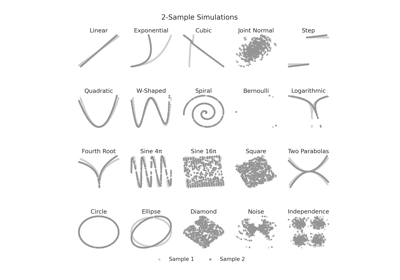# rot_ksamp¶

hyppo.tools.rot_ksamp(sim, n, p, k=2, noise=True, degree=90, pow_type='samp', **kwargs)

Rotates input simulations to produce a k-sample simulation.

Parameters
• sim (str) -- The name of the simulation (from the hyppo.tools module) that is to be rotated.

• n (int) -- The number of samples desired by sim (>= 5).

• p (int) -- The number of dimensions desired by sim (>= 1).

• k (int, default: 2) -- The number of groups to simulate.

• noise (bool, default: True) -- Whether or not to include noise in the simulation.

• degree (float or list of float, default: 90) -- The number of degrees to rotate the input simulation by (in first dimension). The list must be the same size as k - 1.

• pow_type ("samp", "dim", default: "samp") -- Simulation type, (increasing sample size or dimension).

• **kwargs -- Additional keyword arguments for the independence simulation.

Returns

sims (list of ndarray) -- Rotated data matrices. sims is a list of arrays of shape (n, p+1) or (n, 2p) depending on the independence simulation. Here, n is the number of samples and p is the number of dimensions.

## Examples using hyppo.tools.rot_ksamp¶